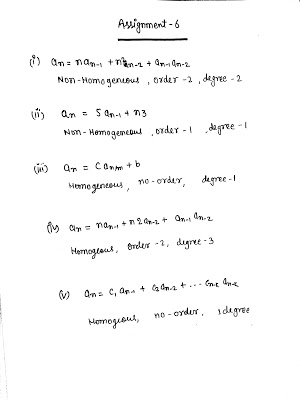Find the order and degree of the following recurrence relation. Also, determine whether they are homogeneous or non-homogeneous. Constant coefficient and non constant coefficient.﻿(i) an = nan-1 + n2﻿ an-2 + an-1 an-2﻿(ii) an = 5 an-1 + n3﻿ (iii) an = C an/m + b﻿ (iv) an = nan-1 + n2an-2 + an-1 an-2﻿ (v) an = C1 an-1 + C2 an-2+ Read More …

Find the order and degree of the following recurrence relation. Also, determine whether they are homogeneous or non-homogeneous. Constant coefficient and non constant coefficient.﻿(i) an = nan-1 + n2﻿ an-2 + an-1 an-2 ﻿(ii) an = 5 an-1 + n3﻿ (iii) an = C an/m + b﻿ (iv) an = nan-1 + n2an-2 + an-1 an-2﻿ (v) an = C1 an-1 + C2 Read More …

Find the order and degree of the following recurrence relation. Also, determine whether they are homogeneous or non-homogeneous. Constant coefficient and non constant coefficient.﻿(i) an = nan-1 + n2﻿ an-2 + an-1 an-2﻿(ii) an = 5 an-1 + n3﻿ (iii) an = C an/m + b﻿ (iv) an = nan-1 + n2an-2 + an-1 an-2﻿ (v) an = C1 an-1 + C2 an-2+ Read More …